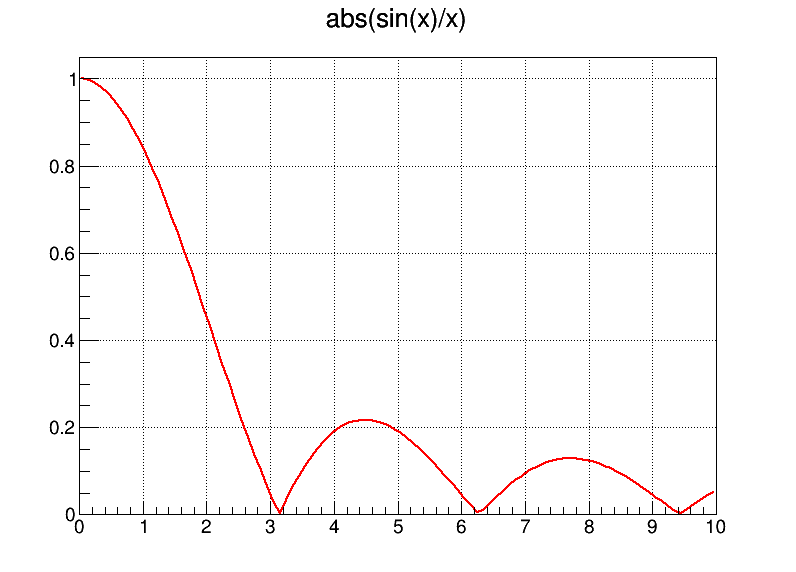In :
import rootnotes
In :
from ROOT import TCanvas, TFormula, TF1
from ROOT import gROOT, gObjectTable
c1 = rootnotes.default_canvas()

We create a formula object and compute the value of this formula for two different values of the x variable.

In :
form1 = TFormula( 'form1', 'sqrt(abs(x))' )
form1.Eval( 2 )
form1.Eval( -45 )
Out:
6.708203932499369

Create a one dimensional function and draw it

In :
fun1 = TF1( 'fun1', 'abs(sin(x)/x)', 0, 10 )
c1.SetGridx()
c1.SetGridy()
fun1.Draw()
c1
Out:In :
canvas = TCanvas("icanvas", "icanvas", 800, 600)
TCanvas::Constructor:0: RuntimeWarning: Deleting canvas with same name: icanvas
In :